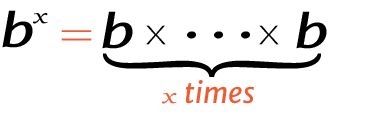# 5 raise to the power of 3

Place the base and exponent in the power calculator to get the right answer for value of 3^5, 35 (3 power 5), or 5 raised to the power of 3.

## How to calculate 3 raise to the power of 5?

This is how you can solve (3) 5 manually.

Solution:

Step 1: Simplify the data.

Base
ExponentStep 2: Multiply the 3 to itself 5 times.

Using this exponents calculator could be much easier to figure out an exponential expression.